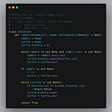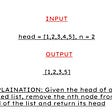# [LeetCode]#1534. Count Good Triplets

• `0 <= i < j < k < arr.length`
• `|arr[i] - arr[j]| <= a`
• `|arr[j] - arr[k]| <= b`
• `|arr[i] - arr[k]| <= c`
`Input: arr = [3,0,1,1,9,7], a = 7, b = 2, c = 3Output: 4Explanation: There are 4 good triplets: [(3,0,1), (3,0,1), (3,1,1), (0,1,1)].`
1. Match three conditions as below.
2. If get 3 passes, return 1 match.
`class Solution:    def countGoodTriplets(self, arr, a, b, c):        ans = 0for i in range(len(arr)):            for j in range(i+1, len(arr)):                for k in range(j+1, len(arr)):                    a_pass = abs(arr[i] - arr[j]) <= a                    b_pass = abs(arr[j] - arr[k]) <= b                    c_pass = abs(arr[i] - arr[k]) <= c                                        if a_pass and b_pass and c_pass:                        ans += 1        return ans`

## More from Fatboy Slim

Interesting in any computer science.

Love podcasts or audiobooks? Learn on the go with our new app.

## Korean Tokenization & Lemmatization## Learning C++: The STL and the List Class## Git for beginners: safe ignorance## Check if Enough Free Space in Remote Computer with PowerShell## Maintenance Announcement## Buildup circuit quickly with ATmega32## Weighted Quick Union algorithm explained## AWS CLI version2 — Hands-On## Fatboy Slim

Interesting in any computer science.

## How to implement a Queue class using deque. PYTHON data structures and algorithm.## [LeetCode]#2057. Smallest Index With Equal Value